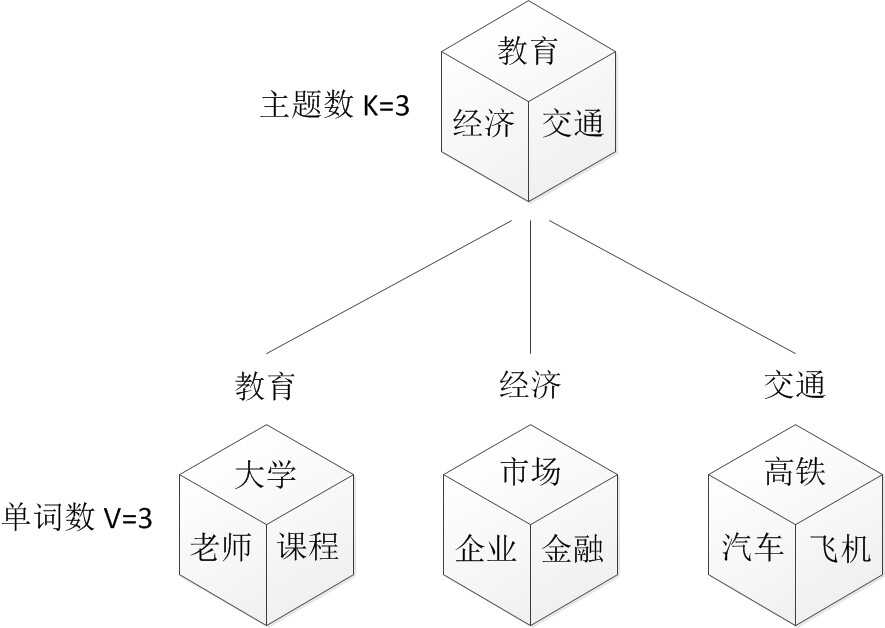# 【机器学习】主题模型

## 1. LDA模型是什么

LDA可以分为以下5个步骤：

• 一个函数：gamma函数。
• 四个分布：二项分布、多项分布、beta分布、Dirichlet分布。
• 一个概念和一个理念：共轭先验和贝叶斯框架。
• 两个模型：pLSA、LDA。
• 一个采样：Gibbs采样• 从狄利克雷分布 $\alpha$ 中取样生成文档 i 的主题分布 $\theta_i$。
• 从主题的多项式分布 $\theta_i$ 中取样生成文档i第 j 个词的主题 $z_{i,j}$。
• 从狄利克雷分布 $\beta$ 中取样生成主题 $z_{i,j}$ 对应的词语分布 $\phi_{z_{i,j}}$。
• 从词语的多项式分布 $\phi_{z_{i,j}}$ 中采样最终生成词语 $w_{i,j}$。### 1.1 5个分布的理解

1. 二项分布（Binomial distribution）

二项分布是从伯努利分布推进的。伯努利分布，又称两点分布或0-1分布，是一个离散型的随机分布，其中的随机变量只有两类取值，非正即负{+，-}。而二项分布即重复n次的伯努利试验，记为 $X\sim_{}b(n,p)$。简言之，只做一次实验，是伯努利分布，重复做了n次，是二项分布。

2. 多项分布

是二项分布扩展到多维的情况。多项分布是指单次试验中的随机变量的取值不再是0-1的，而是有多种离散值可能（1,2,3…,k）。比如投掷6个面的骰子实验，N次实验结果服从K=6的多项分布。其中：

$$\sum_{i=1}^{k}p_i=1,p_i>0$$

3. 共轭先验分布

贝叶斯统计中，如果后验分布先验分布属于同类，则先验分布与后验分布被称为共轭分布，而先验分布被称为似然函数的共轭先验

4. Beta分布

二项分布的共轭先验分布。给定参数 $\alpha>0$ 和 $\beta>0$，取值范围为[0,1]的随机变量 x 的概率密度函数：

$$f(x;\alpha,\beta)=\frac{1}{B(\alpha,\beta)}x^{\alpha-1}(1-x)^{\beta-1}$$

其中：

$$\frac{1}{B(\alpha,\beta)}=\frac{\Gamma(\alpha+\beta)}{\Gamma(\alpha)\Gamma(\beta)}$$

$$\Gamma(z)=\int_{0}^{\infty}t^{z-1}e^{-t}dt$$

注：这便是所谓的gamma函数，下文会具体阐述。

5. 狄利克雷分布

是beta分布在高维度上的推广。Dirichlet分布的的密度函数形式跟beta分布的密度函数如出一辙：

$$f(x_1,x_2,…,x_k;\alpha_1,\alpha_2,…,\alpha_k)=\frac{1}{B(\alpha)}\prod_{i=1}^{k}x_i^{\alpha^i-1}$$

其中

$$B(\alpha)=\frac{\prod_{i=1}^{k}\Gamma(\alpha^i)}{\Gamma(\sum_{i=1}^{k}\alpha^i)},\sum_{}x_i=1$$

至此，我们可以看到二项分布和多项分布很相似，Beta分布和Dirichlet 分布很相似。

• beta分布是二项式分布的共轭先验概率分布：对于非负实数 $\alpha$ 和 $\beta$ ，我们有如下关系：

$$Beta(p|\alpha,\beta)+Count(m_1,m_2)=Beta(p|\alpha+m_1,\beta+m_2)$$

其中 $(m_1,m_2)$ 对应的是二项分布 $B(m_1+m_2,p)$ 的记数。针对于这种观测到的数据符合二项分布，参数的先验分布和后验分布都是Beta分布的情况，就是Beta-Binomial 共轭。”

• 狄利克雷分布（Dirichlet分布）是多项式分布的共轭先验概率分布，一般表达式如下：

$$Dir(\vec{p}|\vec\alpha)+MultCount(\vec{m})=Dir(p|\vec{\alpha}+\vec{m})$$

针对于这种观测到的数据符合多项分布，参数的先验分布和后验分布都是Dirichlet 分布的情况，就是 Dirichlet-Multinomial 共轭。 ”

• 贝叶斯派思考问题的固定模式：

先验分布 $\pi(\theta)$+ 样本信息 $X$ = 后验分布 $\pi(\theta|x)$。

### 1.2 3个基础模型的理解

• $w$ 表示词，$V$ 表示所有单词的个数（固定值）。
• $z$ 表示主题，$k$ 是主题的个数（预先给定，固定值）。
• $D=(W_1,…,W_M)$ 表示语料库，其中的M是语料库中的文档数（固定值）。
• $W=(w_1,w_2,…,w_N)$ 表示文档，其中的N表示一个文档中的词数（随机变量）。
1. Unigram model

对于文档$W=(w_1,w_2,…,w_N)$，用 $p(w_n)$ 表示词 $w_n$ 的先验概率，生成文档w的概率为：

$$p(W)=\prod_{n=1}^{N}p(w_n)$$

2. Mixture of unigrams model

该模型的生成过程是：给某个文档先选择一个主题z,再根据该主题生成文档，该文档中的所有词都来自一个主题。假设主题有 $z_1,…,z_n$，生成文档w的概率为：

$$p(W)=p(z_1)\prod_{n=1}^{N}p(w_n|z_1)+…+p(z_k)\prod_{n=1}^{N}p(w_n|z_k)=\sum_{z}p(z)\prod_{n=1}^{N}p(w_n|z)$$

3. PLSA模型

理解了pLSA模型后，到LDA模型也就一步之遥——给pLSA加上贝叶斯框架，便是LDA。

在上面的Mixture of unigrams model中，我们假定一篇文档只有一个主题生成，可实际中，一篇文章往往有多个主题，只是这多个主题各自在文档中出现的概率大小不一样。比如介绍一个国家的文档中，往往会分别从教育、经济、交通等多个主题进行介绍。那么在pLSA中，文档是怎样被生成的呢？

假定你一共有K个可选的主题，有V个可选的词，咱们来玩一个扔骰子的游戏。

一、假设你每写一篇文档会制作一颗K面的“文档-主题”骰子（扔此骰子能得到K个主题中的任意一个），和K个V面的“主题-词项” 骰子（每个骰子对应一个主题，K个骰子对应之前的K个主题，且骰子的每一面对应要选择的词项，V个面对应着V个可选的词）。

比如可令K=3，即制作1个含有3个主题的“文档-主题”骰子，这3个主题可以是：教育、经济、交通。然后令V = 3，制作3个有着3面的“主题-词项”骰子，其中，教育主题骰子的3个面上的词可以是：大学、老师、课程，经济主题骰子的3个面上的词可以是：市场、企业、金融，交通主题骰子的3个面上的词可以是：高铁、汽车、飞机。二、每写一个词，先扔该“文档-主题”骰子选择主题，得到主题的结果后，使用和主题结果对应的那颗“主题-词项”骰子，扔该骰子选择要写的词。

先扔“文档-主题”的骰子，假设（以一定的概率）得到的主题是教育，所以下一步便是扔教育主题筛子，（以一定的概率）得到教育主题筛子对应的某个词：大学。

上面这个投骰子产生词的过程简化下便是：“先以一定的概率选取主题，再以一定的概率选取词”。

三、最后，你不停的重复扔“文档-主题”骰子和”主题-词项“骰子，重复N次（产生N个词），完成一篇文档，重复这产生一篇文档的方法M次，则完成M篇文档。

上述过程抽象出来即是PLSA的文档生成模型。在这个过程中，我们并未关注词和词之间的出现顺序，所以PLSA是一种词袋方法。生成文档的整个过程便是选定文档生成主题，确定主题生成词。

$$P(w_j|d_i)=\sum_{k=1}^{K}P(w_j|z_k)P(z_k|d_i)$$

$$P(d_i,w_j)=P(d_i)P(w_j|d_i)=P(d_i)\sum_{k=1}^{K}P(w_j|z_k)P(z_k|d_i)$$

### 1.3 LDA模型

• 按照先验概率 $P(d_i)$ 选择一篇文档 $d_i$。
• 从狄利克雷分布（即Dirichlet分布）$\alpha$ 中取样生成文档 $d_i$ 的主题分布 $\theta_i$，换言之，主题分布 $\theta_i$ 由超参数为 $\alpha$ 的Dirichlet分布生成。
• 从主题的多项式分布 $\theta_i$ 中取样生成文档 $d_i$ 第 j 个词的主题 $z_{i,j}$。
• 从狄利克雷分布（即Dirichlet分布）$\beta$ 中取样生成主题 $z_{i,j}$ 对应的词语分布 $\phi_{z_{i,j}}$，换言之，词语分布 $\phi_{z_{i,j}}$ 由参数为 $\beta$ 的Dirichlet分布生成。
• 从词语的多项式分布 $\phi_{z_{i,j}}$ 中采样最终生成词语 $w_{i,j}$。

LDA中，选主题和选词依然都是两个随机的过程，依然可能是先从主题分布{教育：0.5，经济：0.3，交通：0.2}中抽取出主题：教育，然后再从该主题对应的词分布{大学：0.5，老师：0.3，课程：0.2}中抽取出词：大学。

PLSA中，主题分布和词分布是唯一确定的，能明确的指出主题分布可能就是{教育：0.5，经济：0.3，交通：0.2}，词分布可能就是{大学：0.5，老师：0.3，课程：0.2}。

LDA参数估计：Gibbs采样，详见文末的参考文献。

## 2. 怎么确定LDA的topic个数？

1. 基于经验 主观判断、不断调试、操作性强、最为常用。
2. 基于困惑度（主要是比较两个模型之间的好坏）。
3. 使用Log-边际似然函数的方法，这种方法也挺常用的。
4. 非参数方法：Teh提出的基于狄利克雷过程的HDP法。
5. 基于主题之间的相似度：计算主题向量之间的余弦距离，KL距离等。

## 3. 如何用主题模型解决推荐系统中的冷启动问题？

• 用户冷启动是指对一个之前没有行为或行为极少的新用户进行推荐；
• 物品冷启动是指为一个新上市的商品或电影（这时没有与之相关的 评分或用户行为数据）寻找到具有潜在兴趣的用户；
• 系统冷启动是指如何为一个 新开发的网站设计个性化推荐系统。

## 5. 代码实现

LDA模型应用：一眼看穿希拉里的邮件